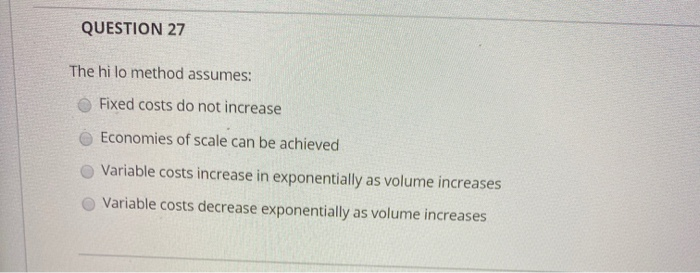# Question Solved1 AnswerQUESTION 27 The hi lo method assumes: Fixed costs do not increase Economies of scale can be achieved Variable costs increase in exponentially as volume increases Variable costs decrease exponentially as volume increasesTranscribed Image Text: QUESTION 27 The hi lo method assumes: Fixed costs do not increase Economies of scale can be achieved Variable costs increase in exponentially as volume increases Variable costs decrease exponentially as volume increases
More
Transcribed Image Text: QUESTION 27 The hi lo method assumes: Fixed costs do not increase Economies of scale can be achieved Variable costs increase in exponentially as volume increases Variable costs decrease exponentially as volume increases